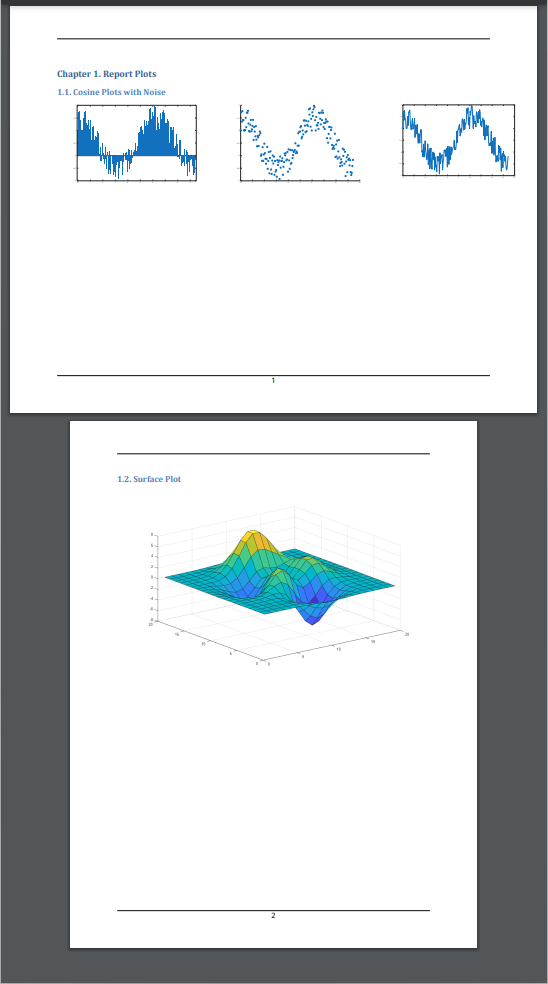# Create a Report With Portrait and Landscape Pages

This example shows how to create a report that contains both 11 x 8.5-in landscape and 8.5 x 11-in portrait pages. The report uses a landscape page to fit content that is too wide to fit on a portrait page, such as these side-by-side images.Import the DOM and Report API packages so you do not have to use long class names.

```import mlreportgen.dom.*; import mlreportgen.report.*;```

Create a container to hold the report content.

```% To create a Word report, change the output type from "pdf" to "docx". rpt = mlreportgen.report.Report("PortraitAndLandscapeReport", "pdf");```

Create a chapter with the title "Types of Cosine Value Plots with Random Noise".

`chapter = Chapter("Title", "Types of Cosine Value Plots with Random Noise");`

Set the chapter landscape layout to true. This will set the entire chapter layout to landscape.

`chapter.Layout.Landscape = true;`

Create the variables to plot. Create x as 200 equally spaced values between 0 and 3pi. Create y as cosine values with random noise.

```x = linspace(0,3*pi,200); y = cos(x) + rand(1,200); ```

Create figure objects of the x and y values: bar graph (fig1), scatter plot (fig2) and 2-D Line plot (fig3) .

Create image objects wrapped around the figure snapshot image files. Set the scaling of the figure objects so they fit in the table entries.

```imgStyle = {ScaleToFit(true)}; fig1 = Figure(bar(x, y)); fig1Img = Image(getSnapshotImage(fig1, rpt)); fig1Img.Style = imgStyle; delete(gcf); fig2 = Figure(scatter(x,y)); fig2Img = Image(getSnapshotImage(fig2, rpt)); fig2Img.Style = imgStyle; delete(gcf); fig3 = Figure(plot(x,y)); fig3Img = Image(getSnapshotImage(fig3, rpt)); fig3Img.Style = imgStyle; delete(gcf);```

Insert the images in the only row of a 1x5 invisible layout table(lo_table)(space between figures by having 2 empty table entries). A table is considered invisible when the borders are not defined for the table nor any of its table entries. The images are sized to fit the table entries only if the height and width of table entries are specified.

```lo_table = Table({fig1Img, ' ', fig2Img, ' ',fig3Img}); lo_table.entry(1,1).Style = {Width('3.2in'), Height('3in')}; lo_table.entry(1,2).Style = {Width('.2in'), Height('3in')}; lo_table.entry(1,3).Style = {Width('3.2in'), Height('3in')}; lo_table.entry(1,4).Style = {Width('.2in'), Height('3in')}; lo_table.entry(1,5).Style = {Width('3in'), Height('3in')};```

Add the table to the chapter and the chapter to the report.

```add(chapter, lo_table); add(rpt, chapter);```

Create a chapter with the title "Surface Plot". The default layout for a chapter is portrait.

`chapter1 = Chapter("Title", "Surface Plot");`

Create a figure object for surface plot (fig4). Create image objects wrapped around the figure snapshot image files.

```fig4 = Figure(surf(peaks(20))); fig4Img = Image(getSnapshotImage(fig4, rpt)); fig4Img.Style = imgStyle; delete(gcf);```

Add the generated image object to the chapter and the chapter to the report.

```add(chapter1, fig4Img); add(rpt, chapter1);```

Generate and display the report

```close(rpt); rptview(rpt);```

The generated report includes the side-by-side figure snapshots on a landscape page and the surface plot on a portrait page.

## SupportGet trial now